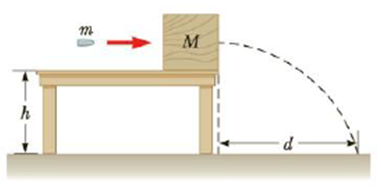Chapter 6, Problem 42P

Chapter
Section
Textbook Problem

An bullet of mass m = 8.00 g is fired into a block of mass M = 250 g that is initially at rest at the edge of a table of height h = 1.00 m (Fig. P6.42). The bullet remains in the block, and after the impact the block lands d = 2.00 m from the bottom of the table. Determine the initial speed of the bullet.Figure P6.42

To determine

Initial speed of the bullet.

Explanation

Given Info: Mass of the bullet is m=8.00×103kg, Mass of the block is M=250×103 kg, initial velocity of the block is vB=0 ,height of the table is Δy=1.00 m, and distance moved by block is Δx=2.00 m.

The expression for projectile motion of the block-bullet combination is

Δy=v0yt+12ayt2                                                                          (1)

• Δy is the height of the table.
• v0y is the vertical speed of the block-bullet combination.
• t is the time taken by  block –bullet combination to reach the floor.
• ay is the acceleration due to gravity.

Substitute 1.00 m for Δy,0 for v0y,9.80 m/s2 for ay in equation (1)

1.00m=(0)t+12(9.80 m/s2)t21.00m=(4.9m/s2)t2t2=1.00m4.9m/s2t=0.452

Thus the time taken by block –bullet combination to reach the floor is 0.452s.

The expression for velocity of the bullet remains in the block is,

v0x=Δxt

• v0x is the horizontal speed of the block-bullet combination

Still sussing out bartleby?

Check out a sample textbook solution.

See a sample solution

The Solution to Your Study Problems

Bartleby provides explanations to thousands of textbook problems written by our experts, many with advanced degrees!

Get Started

In which domain and kingdom are humans classified?

Biology: The Dynamic Science (MindTap Course List)

How does a tendon differ from a ligament?

Human Biology (MindTap Course List)

Complete the following reactions: a. b. c.

Chemistry for Today: General, Organic, and Biochemistry

List the differences between mitosis and meiosis in the following chart:

Human Heredity: Principles and Issues (MindTap Course List)